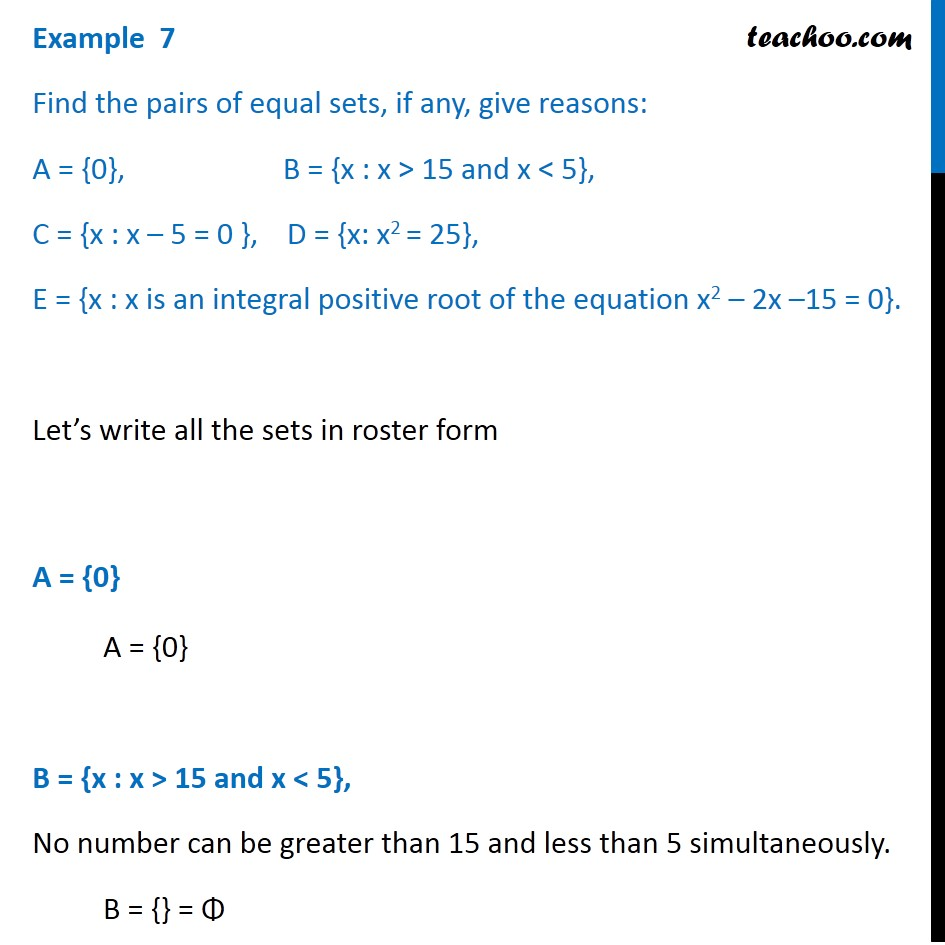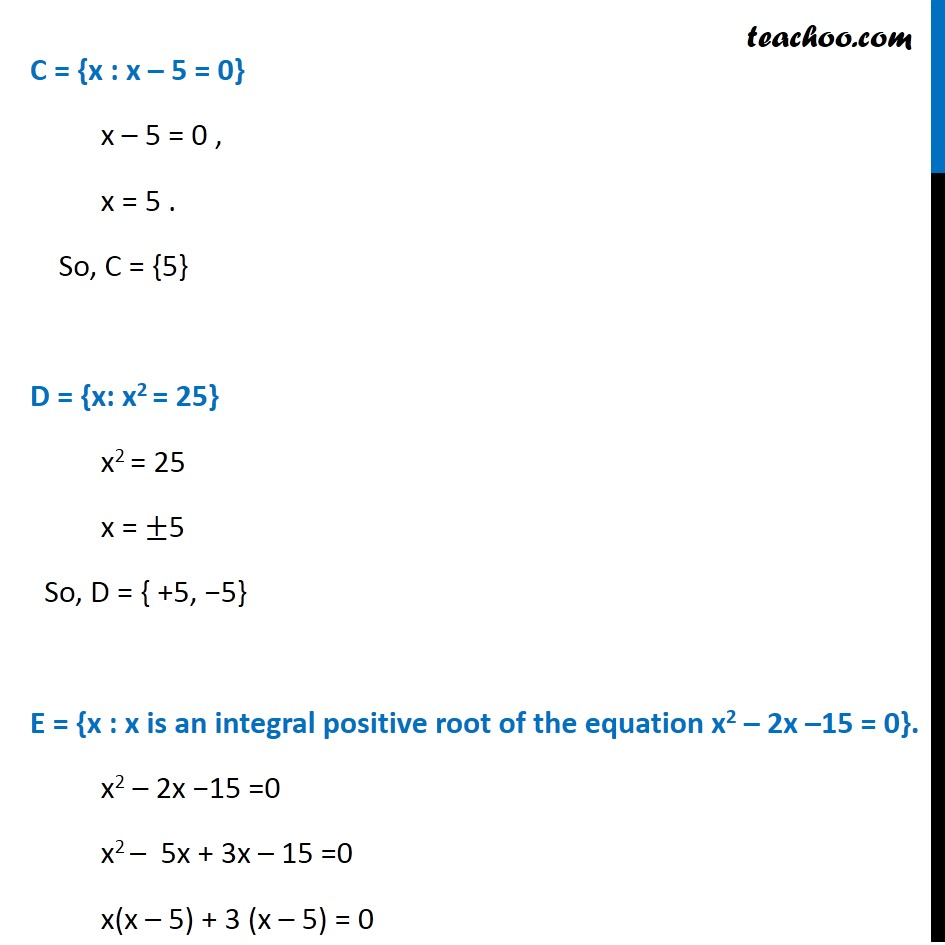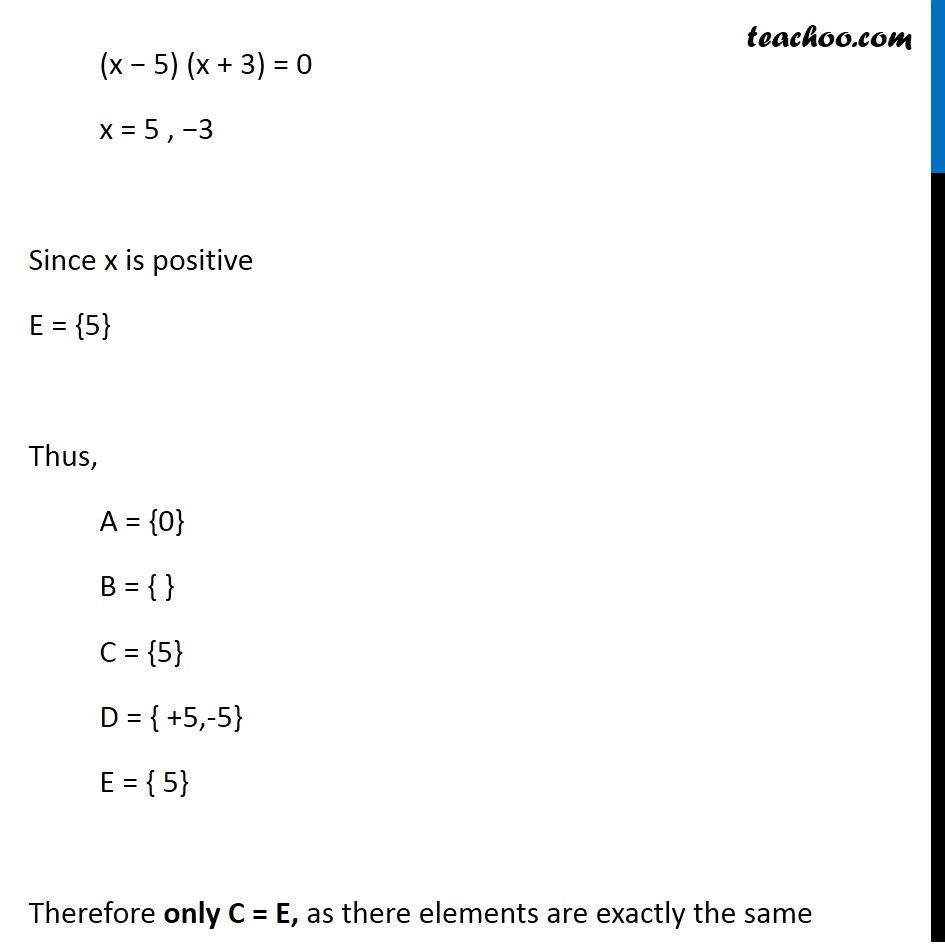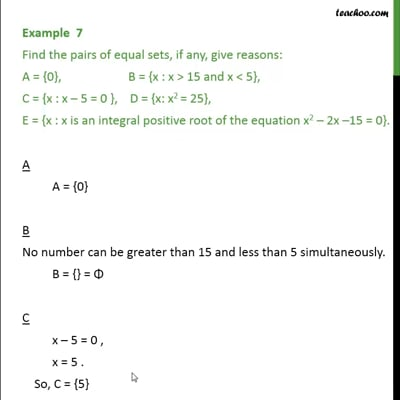Equal sets

Chapter 1 Class 11 Sets
Concept wiseThis video is only available for Teachoo black users

Introducing your new favourite teacher - Teachoo Black, at only ₹83 per month

### Transcript

Example 7 Find the pairs of equal sets, if any, give reasons: A = {0}, B = {x : x > 15 and x < 5}, C = {x : x – 5 = 0 }, D = {x: x2 = 25}, E = {x : x is an integral positive root of the equation x2 – 2x –15 = 0}. Let’s write all the sets in roster form A = {0} A = {0} B = {x : x > 15 and x < 5}, No number can be greater than 15 and less than 5 simultaneously. B = {} = Φ C = {x : x – 5 = 0} x – 5 = 0 , x = 5 . So, C = {5} D = {x: x2 = 25} x2 = 25 x = ±5 So, D = { +5, −5} E = {x : x is an integral positive root of the equation x2 – 2x –15 = 0}. x2 – 2x −15 =0 x2 – 5x + 3x – 15 =0 x(x – 5) + 3 (x – 5) = 0 (x − 5) (x + 3) = 0 x = 5 , −3 Since x is positive E = {5} Thus, A = {0} B = { } C = {5} D = { +5,-5} E = { 5} Therefore only C = E, as there elements are exactly the same